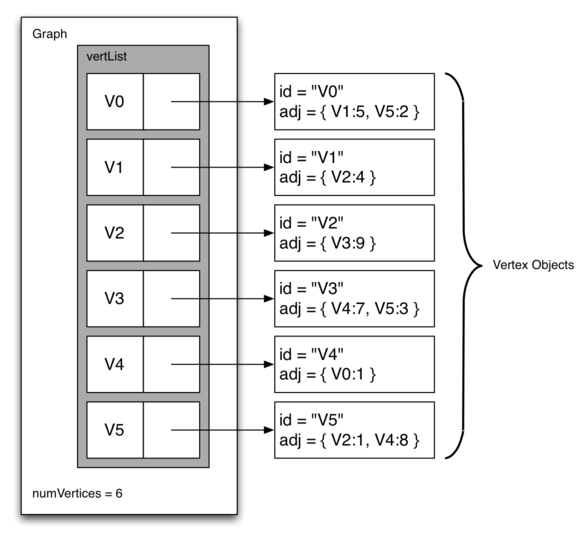A more space-efficient way to implement a sparsely connected graph is to use an adjacency list. In an adjacency list implementation we keep a master list of all the vertices in the Graph object and then each vertex object in the graph maintains a list of the other vertices that it is connected to. In our implementation of the Vertex class we will use a dictionary rather than a list where the dictionary keys are the vertices, and the values are the weights. Figure 4 illustrates the adjacency list representation for the graph in Figure 2.